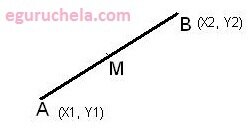### Mid Point Calculator

Calculate the midpoint between two Entered coordinates (x1 , y1 ) and (x2 , y2) in the XY plane by averaging the XY coordinates. The Midpoint Between (x1 , y1 ) and (x2 , y2) points measure a linear midpoint between two locations.
Midpoint Formula: M = ((x1 + x2)/2 , (y1 + y2)/2)X1: Y1: X2: Y2:

 Mid Point
Find the point which is exactly halfway between two given points by averaging the x-values and the y-values. In geometry, the point on a line segment that divides the line into two equal parts.